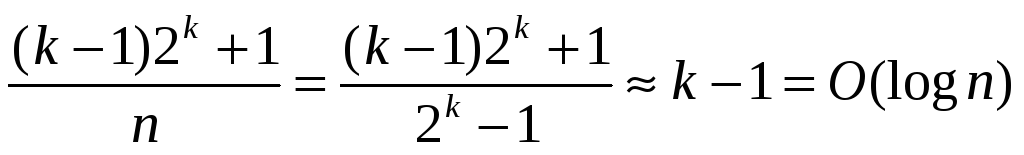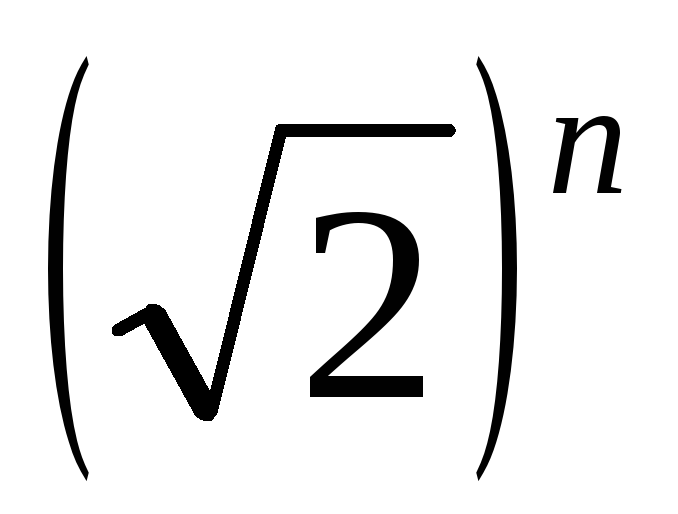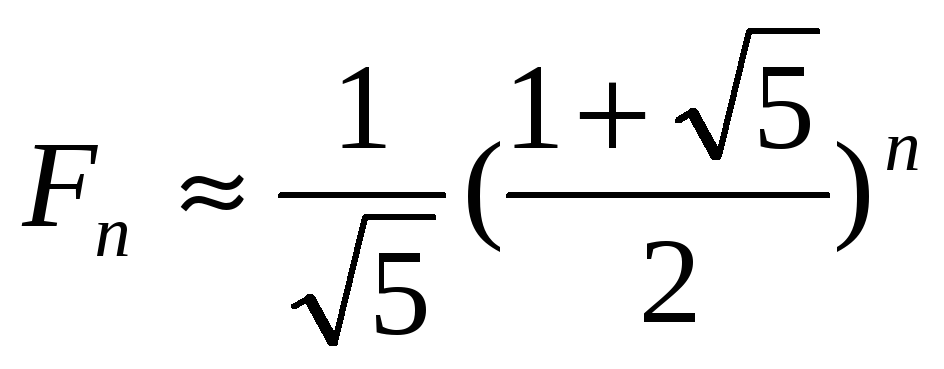# Thus, the average run-time of the binary search is

 Thus, the average run-time of the binary search isSo, for this particular algorithm, the average case run-time is much closer to the worst case run-time than the best-case run time. (Notice that the worst case number of comparisons is k with the average number of comparisons is k-1, where k = log n.) Recurrence Relations and More Analysis A powerful tool in algorithm analysis for recursive methods is recurrence relations. You should have seen some of this in CS1. Let's use the Towers of Hanoi as an example. Let T(n) denote the minimal number of moves it takes to transfer a tower of n disks. We know that T(1) = 1. We also previously made the following observation: In order to move a tower of n disks, we are FORCED to move a tower of n-1 disks, then move the bottom disk, followed by moving a tower of n-1 disks again. Thus we have: T(n)  T(n-1) + 1 + T(n-1), so T(n)  2T(n-1) + 1. But we know we can achieve equality using the method prescribed in class last time, so it follows that T(n) = 2T(n-1) +1, and T(1) = 1. We will use iteration to solve this recurrence relation: T(n) = 2T(n-1) + 1 = 2(2T(n-2) +1) + 1 = 4T(n-2) + 3 = 4(2T(n-3) +1) + 3 = 8T(n-3) + 7 = 2n-1T(1) + 2n-1-1 = 2n-1 + 2n-1-1 = 2(2n-1) - 1 = 2n - 1. Now, let's use induction to verify the result that T(n)=2n-1. Base case: n=1 LHS = T(1) = 1, RHS = 21 - 1 = 1 Inductive hypothesis: Assume for an arbitrary value of n=k that T(k) = 2k - 1 Inductive step: Prove that for n=k+1 that T(k+1)=2k+1-1. T(k+1) = 2T(k) + 1, using the given recurrence = 2(2k - 1) + 1, using the inductive hypothesis = 2k+1 - 2 + 1 = 2k+1 - 1, and the proof is finished. The Change Problem "The Change Store" was an old SNL skit (a pretty dumb one...) where they would say things like, "You need change for a 20? We'll give you two tens, or a ten and two fives, or four fives, etc." If you are a dorky minded CS 2 student, you might ask yourself (after you ask yourself why those writers get paid so much for writing the crap that they do), "Given a certain amount of money, how many different ways are there to make change for that amount of money?" Let us simplify the problem as follows: Given a positive integer n, how many ways can we make change for n cents using pennies, nickels, dimes and quarters? Recursively, we could break down the problem as follows: To make change for n cents we could: 1) Give the customer a quarter. Then we have to make change for n-25 cents 2) Give the customer a dime. Then we have to make change for n-10 cents 3) Give the customer a nickel. Then we have to make change for n-5 cents 4) Give the customer a penny. Then we have to make change for n-1 cents. If we let T(n) = number of ways to make change for n cents, we get the formula T(n) = T(n-25)+T(n-10)+T(n-5)+T(n-1) Is there anything wrong with this? If you plug in the initial condition T(1) = 1, T(0)=1, T(n)=0 if n<0, you'll find that the values this formula produces are incorrect. (In particular, for this recurrence relation T(6)=3, but in actuality, we want T(6)=2.) So this can not be right. What is wrong with our logic? In particular, it can been seen that this formula is an OVERESTIMATE of the actual value. Specifically, this counts certain combinations multiple times. In the above example, the one penny, one nickel combination is counted twice. Why is this the case? The problem is that we are counting all combinations of coins that can be given out where ORDER matters. (We are counting giving a penny then a nickel separately from giving a nickel and then a penny.) We have to find a way to NOT do this. One way to do this is IMPOSE an order on the way the coins are given. We could do this by saying that coins must be given from most value to least value. Thus, if you "gave" a nickel, afterwards, you would only be allowed to give nickels and pennies. Using this idea, we need to adjust the format of our recursive computation: To make change for n cents using the largest coin d, we could 1)If d is 25, give out a quarter and make change for n-25 cents using the largest coin as a quarter. 2)If d is 10, give out a dime and make change for n-10 cents using the largest coin as a dime. 3)If d is 5, give out a nickel and make change for n-5 cents using the largest coin as a nickel. 4)If d is 1, we can simply return 1 since if you are only allowed to give pennies, you can only make change in one way. Although this seems quite a bit more complex than before, the code itself isn't so long. Let's take a look at it: public static int makeChange(int n, int d) { if (n < 0) return 0; else if (n==0) return 1; else { int sum = 0; switch (d) { case 25: sum+=makeChange(n-25,25); case 10: sum+=makeChange(n-10,10); case 5: sum += makeChange(n-5,5); case 1: sum++; } return sum; } } Dangers of Recursion In the code above, a whole bunch of stuff going on, but one of the things you'll notice is that the larger n gets, the slower and slower this will run, or maybe your computer will run out of stack space. Further analysis will show that many, many method calls get repeated in the course of a single initial method call. This is the main inefficiency of recursion, namely that method calls that have already been computed get recomputed. To most easily recognize this issue, let's first look at the recursive version of determining the nth Fibonacci number: public static int fibrec(int n) { if (n < 2) return n; else return fibrec(n-1)+fibrec(n-2); } The problem here is that lots of calls are made to fibrec(0) and fibrec(1). More concretely, consider what happens when we call fibrec(10). It calls fibrec(9), which calls fibrec(8) and fibrec(7). When both of those calls finish, we call fibrec(8) AGAIN, even though we had already done that!!! Another way of looking at it is that all answers returned are either 0 or 1, thus the number of recursive calls made is greater than or equal to Fn, the nth Fibonacci number. Now, we will show that F2n  2n, using induction on n for all positive integers n  3. Base case n=3: LHS = F6 = 8, RHS = 23 = 8, so the inequality holds. Inductive hypothesis: Assume for an arbitrary value of n=k that F2k  2k. Inductive step: Prove for n=k+1 that F2(k+1)  2k+1. F2(k+1) = F2k+2 = F2k+1 + F2k > 2F2k, since the sequence is strictly increasing for k>1.  2(2k) = 2k+1, finishing the induction. Basically, this proves that Fn , which in turn proves that the recursive method to solve this problem runs in exponential time in the value of the input parameter. More accurately,, which also indicates the method's running time. Similarly in the change problem, we may very well recursively find out the number of ways to make change for 120 cents multiple times, instead of reusing the answer we computed the first time.Download 246.04 Kb.Share with your friends: